# Model 1 for reference, draw the structure of the given amino acid as it nly exist under each of t...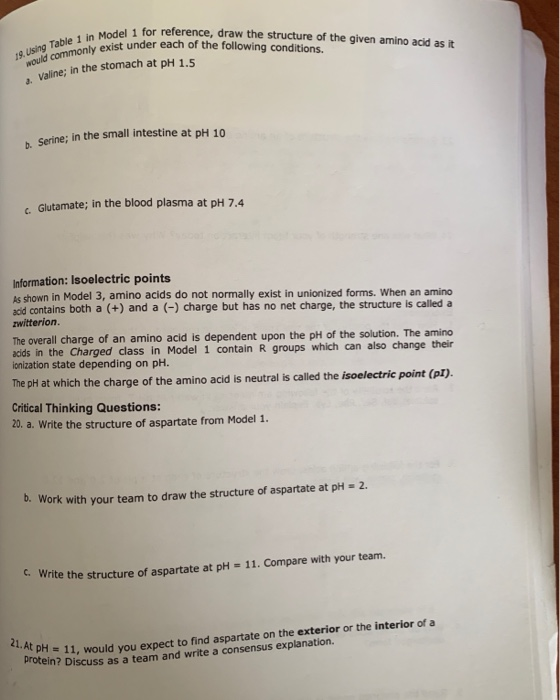Model 1 for reference, draw the structure of the given amino acid as it nly exist under each of the following conditions for 1g.using Table 1 in a. valine; in the stomach at PH 1.5 Serine; in the small intestine at pH 10 c. Glutamate; in the blood plasma at pH 7.4 Information: Isoelectric points As shown in Model 3, amino acids do not normally exist in unionized forms. When an aminc acd contains both a (+) and a () charge but has no net charge, the structure is called a zwitterion The overall charge of an amino acid is dependent upon the pH of the solution. The amino acds in the Charged class in Model 1 contain R groups which can also change their ionization state depending on pH. The pHi at which the charge of the amino acid is neutral is called the isoelectric point (pI) Critical Thinking Questions: 20. a. Write the structure of aspartate from Model 1. b. Work with your team to draw the structure of aspartate at pH 2 c. Write the structure of aspartate at pH - 11. Compare with your team. = 11, would you expect to find aspartate on the exterior or the interior of a n? Discuss as a team and write a consensus explanation. 21. At PH-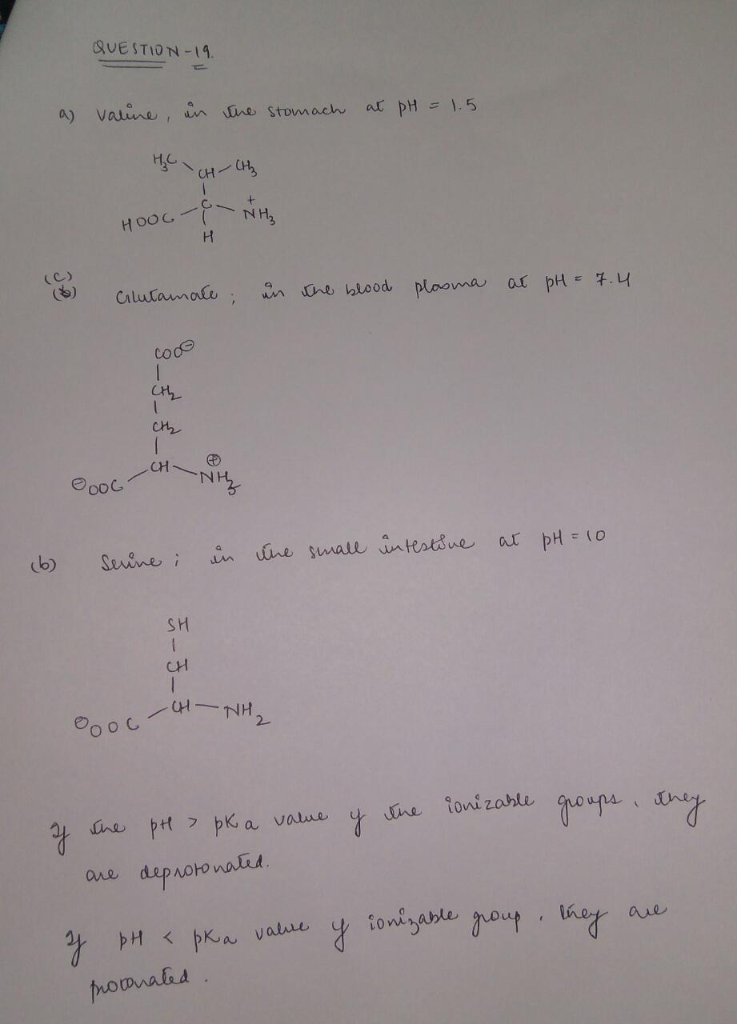##### Add Answer of: Model 1 for reference, draw the structure of the given amino acid as it nly exist under each of t...
Similar Homework Help Questions
• ### 2. Using the reference pk values provided below (assuming that pk values can be applied to...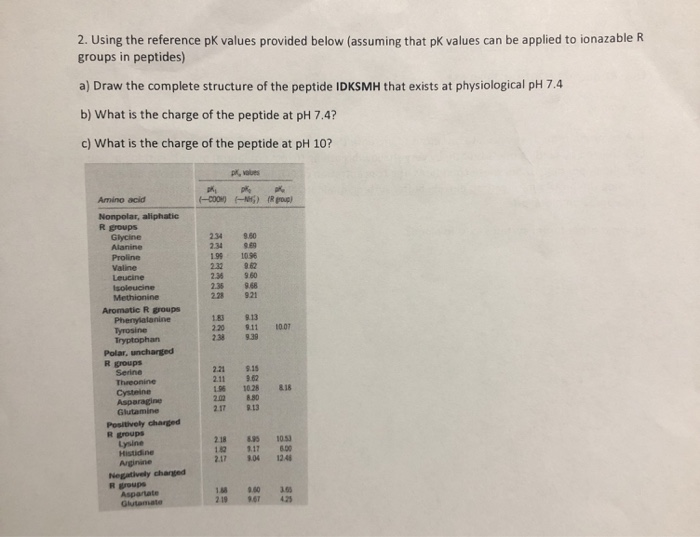2. Using the reference pk values provided below (assuming that pk values can be applied to ionazable R groups in peptides) a) Draw the complete structure of the peptide IDKSMH that exists at physiological pH 7.4 b) What is the charge of the peptide at pH 7.4? c) What is the charge of the peptide at pH 10? PR, es pkk ) Rougl Amino acid COOH) Nonpolar, aliphatic Rgroups Glycine Alanine Proline Valine 1.99 10.98 Loucine 236 2.35 921 8.13...

• ### 1. The pKa's of the amino acid methionine are 2.28 and 9.21. The zwitterion of methionine...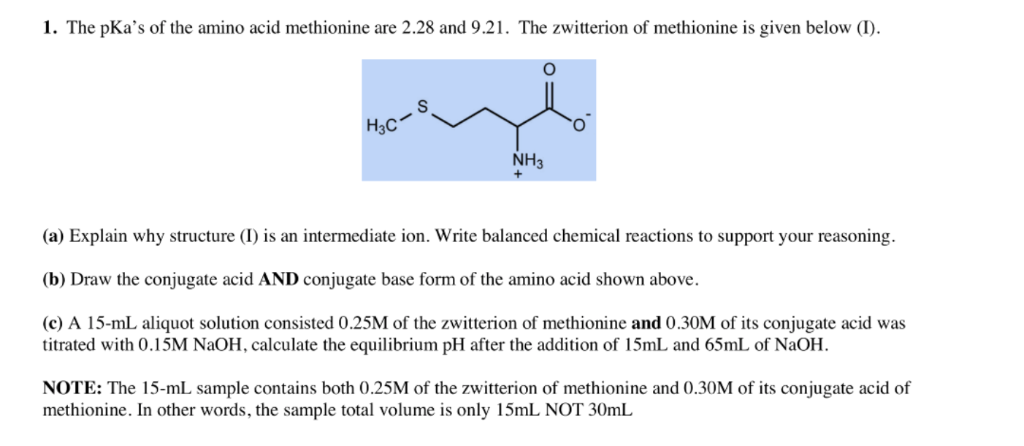1. The pKa's of the amino acid methionine are 2.28 and 9.21. The zwitterion of methionine is given below (1). S Н,с NH3 (a) Explain why structure (I) is an intermediate ion. Write balanced chemical reactions to support your reasoning (b) Draw the conjugate acid AND conjugate base form of the amino acid shown above (c) A 15-mL aliquot solution consisted 0.25M of the zwitterion of methionine and 0.30M of its conjugate acid was titrated with 0.15M NaOH, calculate the...

• ### 1. The pKa's of the amino acid methionine are 2.28 and 9.21. The zwitterion of methionine is given below (I) S H3C...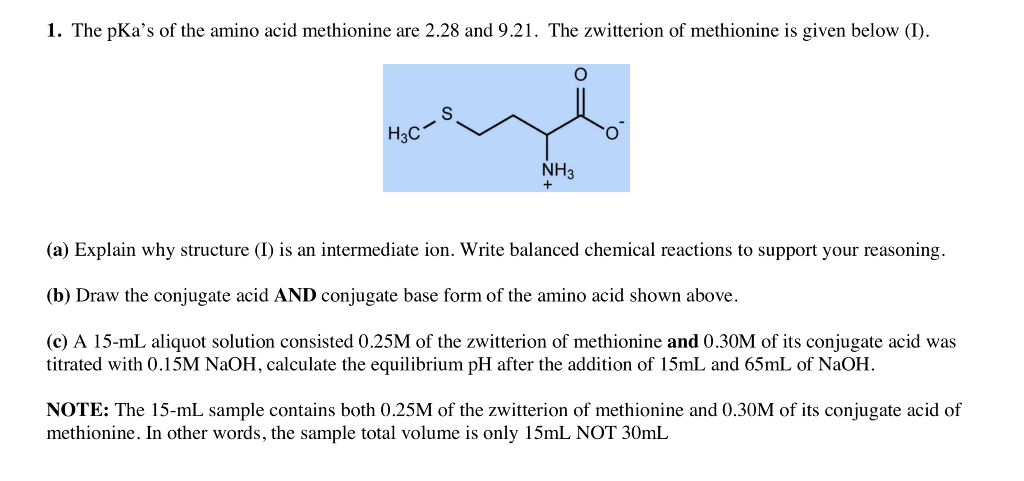1. The pKa's of the amino acid methionine are 2.28 and 9.21. The zwitterion of methionine is given below (I) S H3C O NH3 (a) Explain why structure (I) is an intermediate ion. Write balanced chemical reactions to support your reasoning. (b) Draw the conjugate acid AND conjugate base form of the amino acid shown above (c) A 15-mL aliquot solution consisted 0.25M of the zwitterion of methionine and 0.30M of its conjugate acid was titrated with 0.15M N2OH, calculate...

• ### 2. (10 points) The structure for the amino acid histidine is shown below (at pH 1)....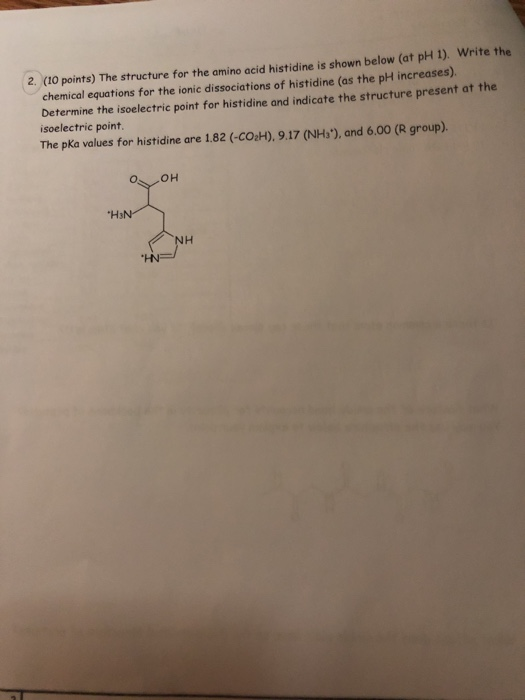2. (10 points) The structure for the amino acid histidine is shown below (at pH 1). Write the chemical equations for the ionic dissociations of histidine (as the pH increases) Determine the isoelectric point for histidine and indicate the structure present at the isoelectric point. The pka values for histidine are 1.82 (-CO2H). 9.17 (NH3'), and 6.00 (R group). OOH "HaN NH "INP

• ### Post-Lab Questions 1. Look up the structure in your textbook of the amino acid you titrated....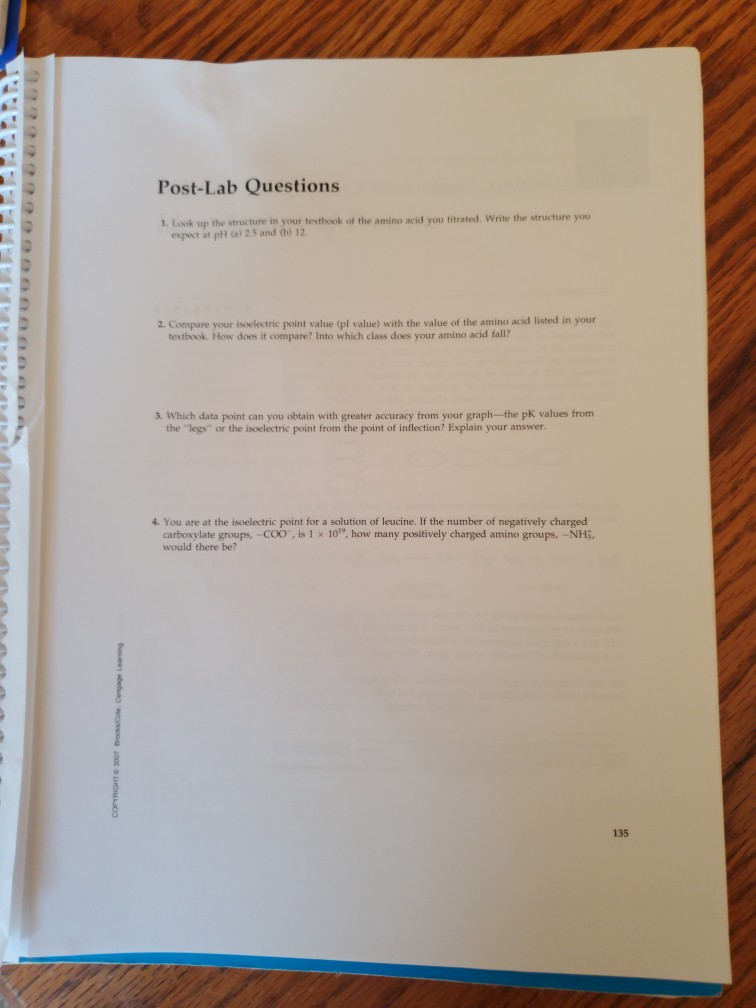Post-Lab Questions 1. Look up the structure in your textbook of the amino acid you titrated. Write the structure you expect at pH (a) 2.5 and (b) 12 2. Compare your isoelectric point value (pl value) with the value of the amino acid listed in your textbook. How does it compare? Into which class does your amino acid fall? 3. Which data point can you obtain with greater accuracy from your graph-the pK values from the "legs" or the isoelectric...

• ### 5. D-sorbital is found in 'sugar-free' chewing gum. It is formed by reducing D-glucose with NaBH Draw the structure of D-sorbital. s. (4 pts) Classify the following amino acid as polar, n...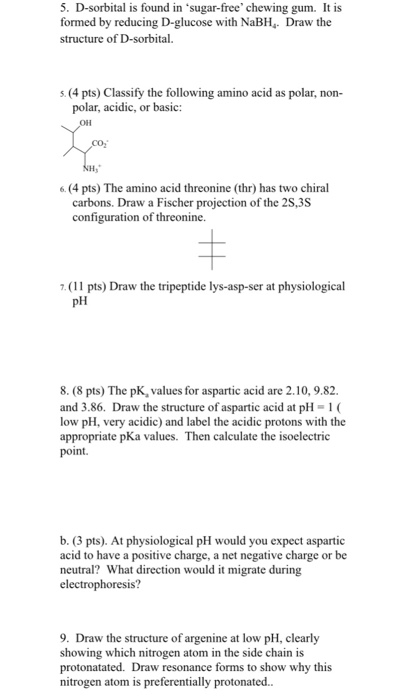5. D-sorbital is found in 'sugar-free' chewing gum. It is formed by reducing D-glucose with NaBH Draw the structure of D-sorbital. s. (4 pts) Classify the following amino acid as polar, non- polar, acidic, or basic: 6 (4 pts) The amino acid threonine (thr) has two chiral carbons. Draw a Fischer projection of the 2S,3S configuration of threonine. キ 7.(11 pts) Draw the tripeptide lys-asp-ser at physiological pH 8. (8 pts) The pK, values for aspartic acid are 2.10, 9.82...

• ### 1.) Draw the structure of the amino acid Serine(ser), which has R=-CH2-OH. Label the alpha carbon. 2.) Draw the dipeptid...

1.) Draw the structure of the amino acid Serine(ser), which has R=-CH2-OH. Label the alpha carbon. 2.) Draw the dipeptide that forms between serine and alanine. The dipeptide sequence is Ser-Ala. Label the peptide bond. 3.) How many amino acids residues are in a polypeptide containing 6 peptide bonds? How many peptide bonds are in a tripeptide 4.) Sketch a alpha-helix and a beta-sheet as best as you can. Label each.

• ### PRELAB ASSIGNMENT 1 Draw the structure of the amino acid son CH-OH Label the alpha carbon...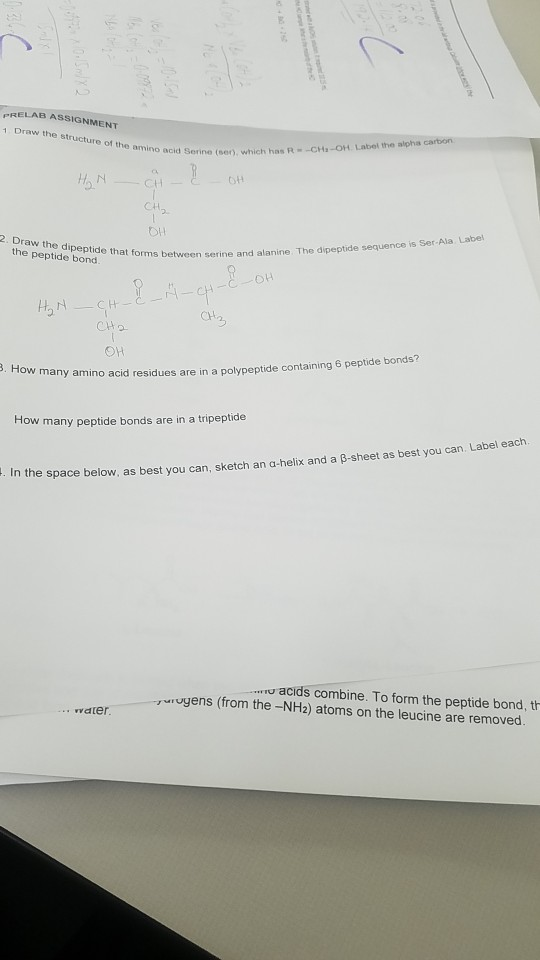PRELAB ASSIGNMENT 1 Draw the structure of the amino acid son CH-OH Label the alpha carbon acid Sorine (en) which has R MO CHE OH CH2 OH 2. Draw the dipeptide that forms betw the peptide bond. s between serine and alanine The dipeptide sequence is Ser Ala Lace CH NCH H, A - — сH — с CH2 OH 3. How many amino aci arty amino acid residues are in a polypeptide containing 6 peptide bonos How many peptide...

• ### 26. Which of the following classification does not match the amino acid side chain A) Contains an basic group/ lysi...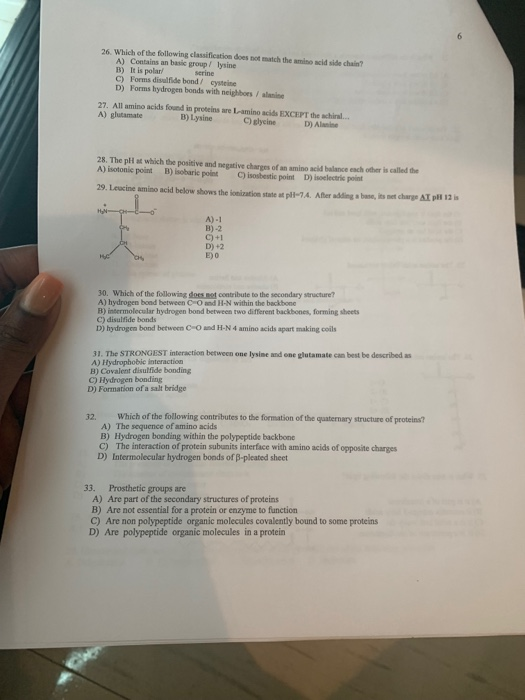26. Which of the following classification does not match the amino acid side chain A) Contains an basic group/ lysine B) It is polar C) Forms disulfide bond/ cysteine D) Forms hydrogen bonds with neighbors/ alanine serine 27. All amino acids found in proteins are L-amino acids EXCEPT the achiral. A) glutamate B) Lysine C) glyeine D) Alamine 28. The plH at which the positive and negative charges of an amino acid balance each ofher is called the A) isotonic...

• ### Please help with 4-10! DNA, Genes,and Protein Synthesis Activity 13: 2. The bases that interact with each other are called complementary bases. this definition and your answers to 1 complete th...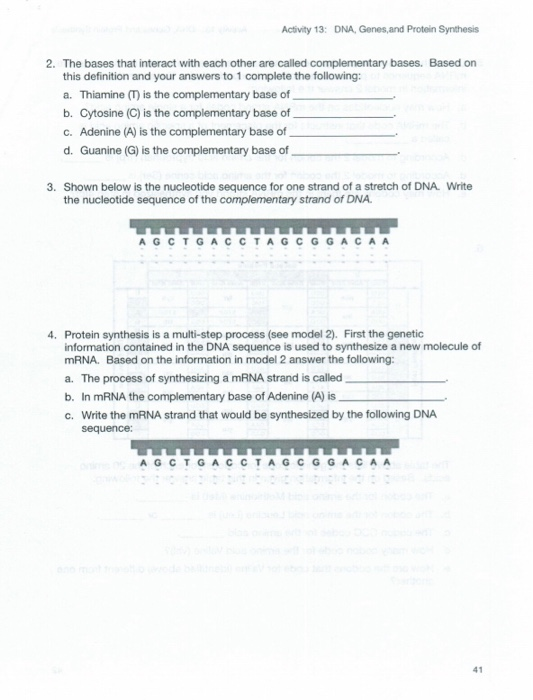Please help with 4-10! DNA, Genes,and Protein Synthesis Activity 13: 2. The bases that interact with each other are called complementary bases. this definition and your answers to 1 complete the following: a. Thiamine (T) is the complementary base of b. Cytosine (C) is the complementary base of c. Adenine (A) is the complementary base of d. Guanine (G) is the complementary base of Based on 3. Shown below is the nucleotide sequence for one strand of a stretch of...

Need Online Homework Help?